# New to Qlik Sense

If you’re new to Qlik Sense, start with this Discussion Board and get up-to-speed quickly.

Announcements
QlikWorld 2023, a live, in-person thrill ride. Save \$300 before February 6: REGISTER NOW!
cancel
Showing results for
Did you mean:MVP

## Creating Mini Charts in Qlik Sense is possible.

Hi all,

Mini Charts has always been a good feature in QlikView and It's really 'frustrating' that this has not been added (yet) in Qlik Sense.

With hopes that the Qlik Team will think about such improvements for the next releases, let's discuss here how to create our own mini chart in a Qlik Sense Table.

Suppose we have:

As a dimension: OICA Region

As measures:

Sum(Sales)

Sum("Commercial Vehicle Sales")

Sum(Sales) / (Sum(Sales)+Sum("Commercial Vehicle Sales"))

Here is our current table: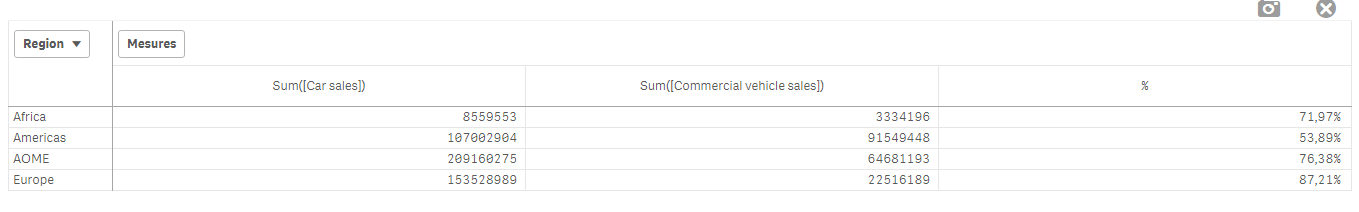Now, we want to add, a mini 'bar' to represent the %:

How to do so:

As an expression:

=repeat('█',

rangemax(rangemin( ceil(((column(1)/column(2))-1)*2.5),10),0)

)

With that, we repeat the square sign and precise the limits.

And as a text expression:

if( (sum([Car sales])/(sum([Car sales])+sum([Commercial vehicle sales])))>=0.8,Green(),

if((sum([Car sales])/(sum([Car sales])+sum([Commercial vehicle sales])))>=0.7,Yellow(),

Red()))

Result: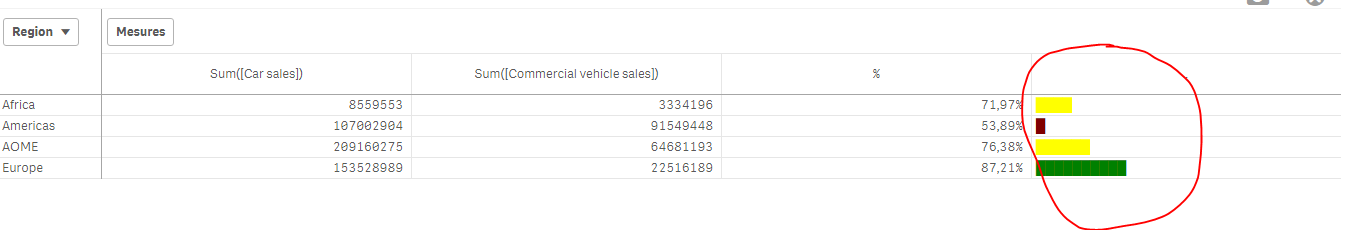We can also Add the % in front of the bar by adding it in the expression:

From :

=repeat('█',

rangemax(rangemin( ceil(((column(1)/column(2))-1)*2.5),10),0)

)

To:

=repeat('█',

rangemax(rangemin( ceil(((column(1)/column(2))-1)*2.5),10),0)

) & num((sum([Car sales])/(sum([Car sales])+sum([Commercial vehicle sales]))),'# ##0,00%')

Result: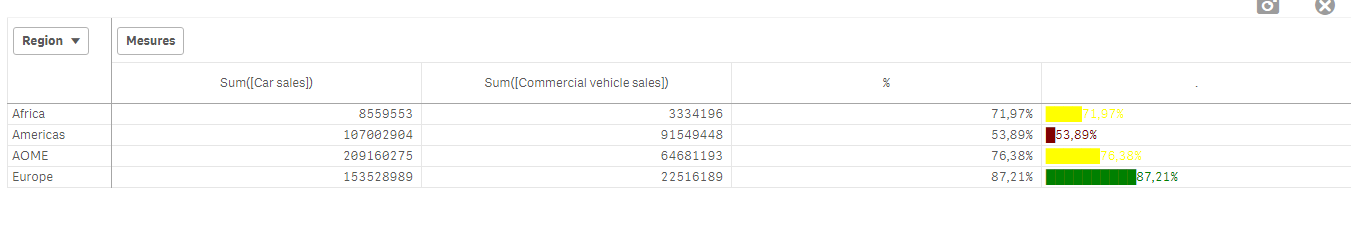Now, let's suppose we want to add another mini chart, a trend one, to see the % expression by Year:

If it has increased or decreased from a year to the next one.

How to do so?

Expression:

=concat(

aggr(

if((sum([Car sales])/(sum([Car sales])+sum([Commercial vehicle sales]))) > above((sum([Car sales])/(sum([Car sales])+sum([Commercial vehicle sales])))),

'▀',if((sum([Car sales])/(sum([Car sales])+sum([Commercial vehicle sales]))) < above((sum([Car sales])/(sum([Car sales])+sum([Commercial vehicle sales])))),'▄',' ')

)

,[OICA region], (Year,(NUMERIC, ASCENDING))

)

,'',[OICA region])

Wirh:

Red: Our Dimension

Green: The trending dimension

Blue: our expression compared to the previous one (by year, the trending expression)

If expression by Year > expression Previous Year then , Square Up

If expression by Year < expression Previous Year then , Square down

Result: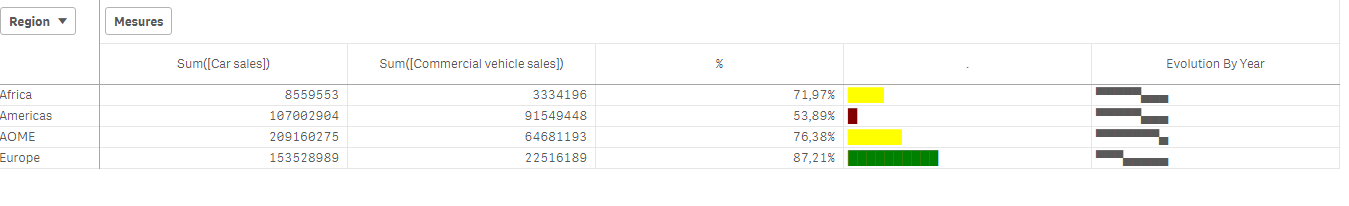Special thanks to blog Von Heldendaten in which I found this:

QlikView + Qlik Sense Blog von Heldendaten: Qlik Sense Calendar Measures & "Minicharts" in Tabellen.

Hope you like it !

Omar,

4 RepliesMVP

Great work omarbensalem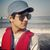Specialist

That's Amazing @OmarBenSalemContributor

Since we Round off the Expression and obtain an Integer, and multiplying it by 10 makes it to show change in the step of 10.

Like 11, 14 and 19 will have the same size of Bar chart. I am having some data to display with accurate figures in a concise manner as their is very narrow changes in the consecutive rows.

Like 42, 45, 46. So I have to multiply by 100 (to get the accurate figure)

Please help to find a workaround on How to make it concise yet accurate. in this particular case.Contributor

I am having some data to display with accurate figures in a concise manner as their is very narrow changes in the consecutive rows.

Like 42, 45, 46. So I have to multiply by 100 (to get the accurate figure and size of the bar)

But doing this doesn't looks good from visualization's perspective.

Please help to find a workaround on How to make it concise yet accurate. in this particular case.Tags
Community Browser